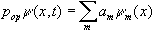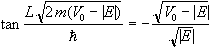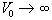# 1.[4pt]Momentum operator in energy representation

For the infinitely deep square well evaluate the momentum operator acting on any wavefunction that solves the Schrödinger equation can be written as.  Here, ym(x) are the stationary solutions to Schrödinger equation.  Evaluate the coefficients am explicitely.

# 2.[3+2pt]Infinite square well in three representations

a)                  Ohanian #9, p.94 (Evaluate the Fourier transform explicitly).

b)                  Plot the functions |y(x)|2 and |f(p)|2 in suitable intervals.

# 3.[4+2pt]Finite square well

a)                  Derive the solutions to the time-independent Schrödinger equation for the finite square well described by the potential in eq. (77) in Ohanian.  For the region |x|<L choose a solution that is proportional to sin(ax) and show that the energy eigenvalues are determined by solutions to.

b)                  Show that in the limitthe expressionreduces to the energy quantization condition for the infinite square well.